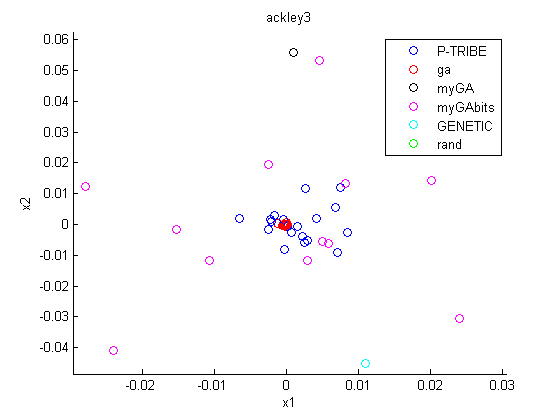MATLAB Global Optimization

A lot of people spend a lot of time to try to figure out to write clever global optimization algorithms. So it won't be easy, but my goal is to eventually figure out a way to write a Global Optimizer that can beat MATLAB's built in Genetic Algorithm -> ga(). I have written my own simple versions of a real-value GA, a bit-string GA, and a Particle Swarm (modeled after TRIBES) algorithm. So far there are some situations where my TRIBES can beat MATLAB's GA, however I still have a long way to go. In most situations none of my algorithms come anywhere close to MATLAB's GA as the number of variables increases.

Put simply the goal of these algorithms is to find the minimum value of a function within a certain allowable range for the variables. After each algorithm is done running it outputs the lowest function value it can find and the point at which it finds this function value. In this graph you can see that my P-TRIBE algorithm seems to be outperforming (the minimum is f=0) everything else even at 10 variables. There is one run (run 44) where MATLABs GA finds a lower minimum than my P-TRIBEs ever found, but in general for any given run the P-TRIBEs has everything else beat.

Ackley Function

This is a surface plot of a 2-variable Ackley function. This plot shows a lower bound of 0 and an upper bound of 10. For the actual testing that will be shown below I used the bounds of -5 to 5.

ackley'n' --> f minimum = 0, x optimal = [0,0,0...]
ackleypos'n' --> f minimum = 0, x optimal = [2,2,2...]
ackleyneg'n' --> f minimum = 0, x optimal = [-2,-2,-2...]
where 'n' is the number of variables.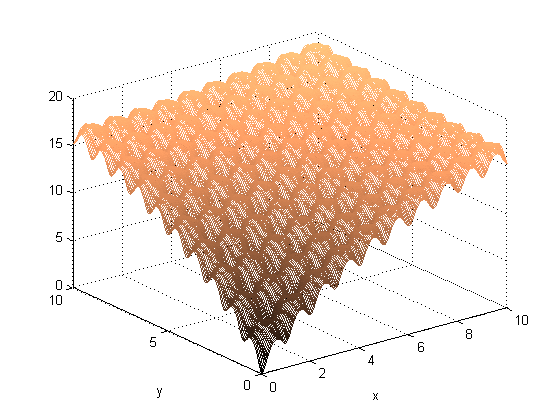These plots show the minimum f value found (y axis) within 1500 function calls over 50 runs (x axis)

Both my P-TRIBE and MATLAB's GA (labeled 'ga' in the legend) are so close to zero every time that you can barely see them. My GAbits also performs relatively well, it may be finding the right area but just needs a little bit of tweaking to zero in on the correct value.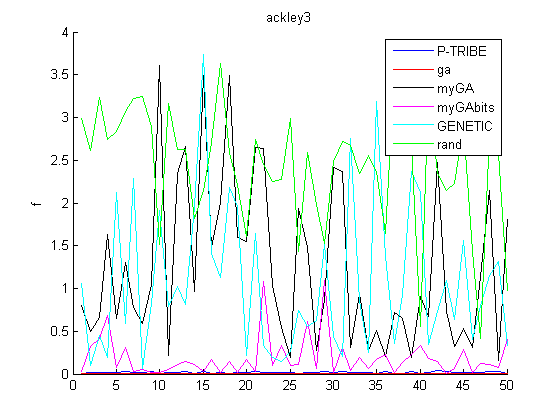Now that the optimum point has moved away from zero MATLABs GA seems to be tripping up and sometimes falling into a local minima and my P-TRIBE stays steadily near zero.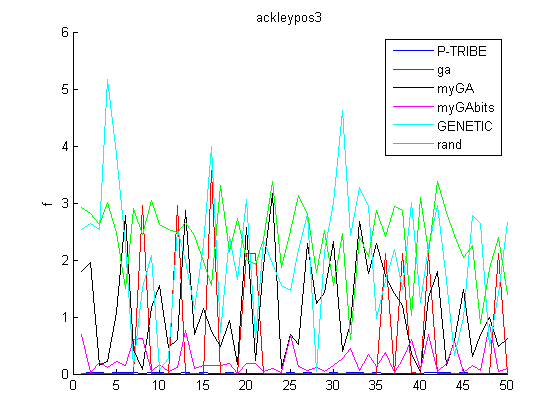Now that the optimum point has become negative MATLABs GA does significantly worse than random guessing ('rand' on the legend) and my P-TRIBE still stays near zero. I'm not sure why this causes problems with MATLABs GA.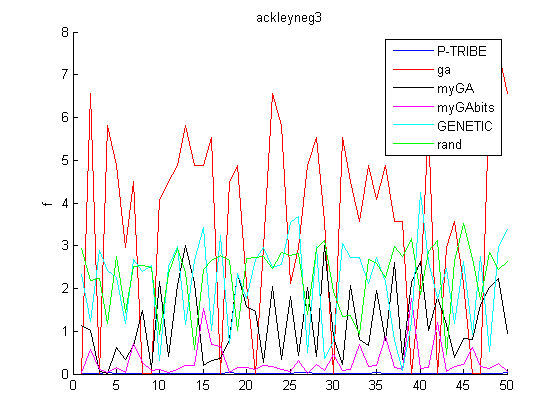Now the number of variables has gone up from 3 to 10 and you can clearly see now how dominant MATLABs GA is.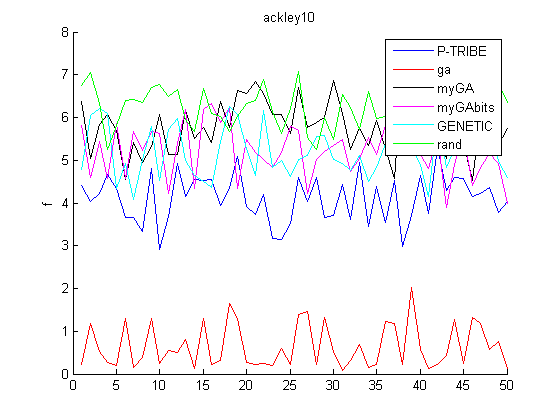Pretty similar to the above. It seems like P-TRIBEs is still slightly better than all of my other algorithms though.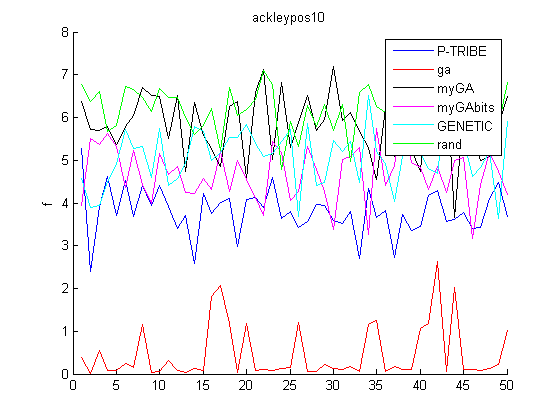MATLABs GA doesn't do as well with the optimum point now back in the negatives. My P-TRIBEs is more successful but nowhere near the true f minimum of zero.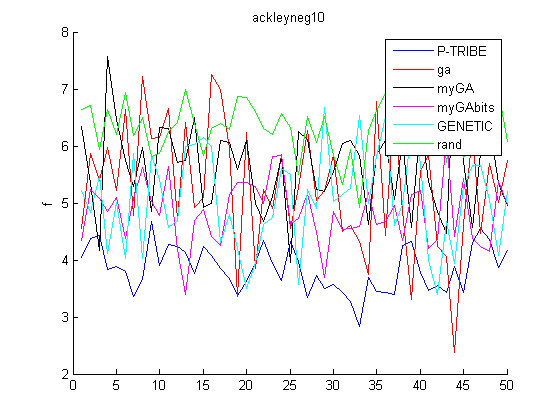Michalewicz Function

Here is a 2 variable Michalewicz function surface plot. I would expect this type of function to possibly favor GAs (especially real-value GAs) over Particle Swarms.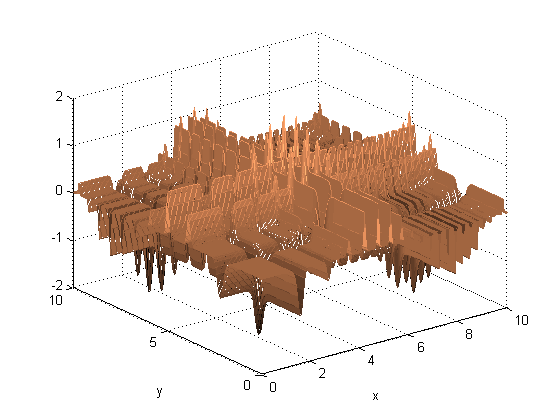With 3 variables MATLABs GA gets a fairly consistent minimum but sometimes my P-TRIBE and GAbits find better solutions. These solutions are very close to the outer edges of the bounded region.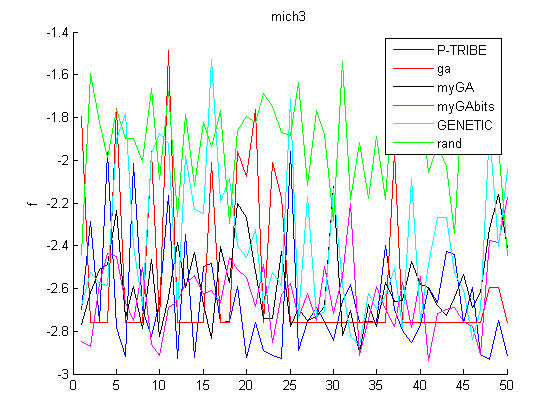Once again at 10 variables MATLABs GA is the clear winner but my real-value GA ('myGA' on the legend) isn't very far off. This makes sense due to the nature of this function having many straight lines along the unit axes.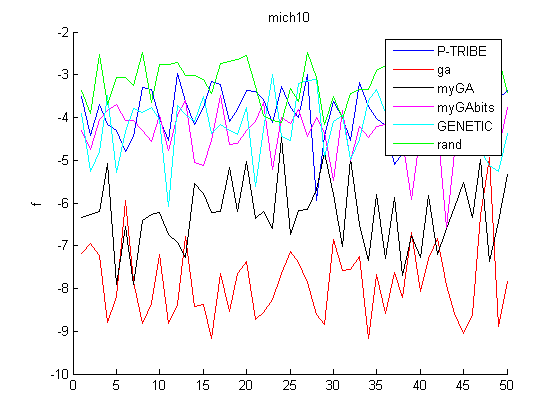Corrugated Spring Function

The last function to be tested was the 'corrugated spring function' shown here with bounds of -5 to 10, optimal solution at [5,5] and minimum function value of -1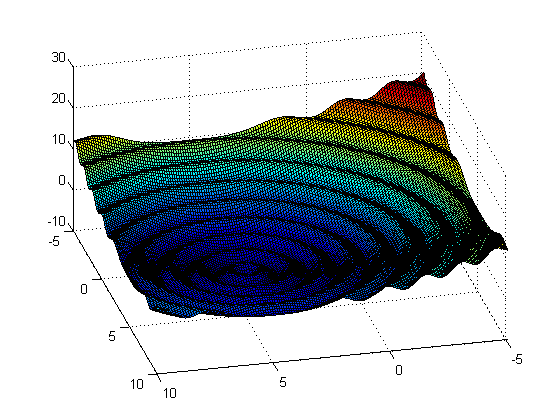With the bounds at -5 to 5 the global minimum of [5,5,5,...] would be right on the edge of the bounds. Some optimizers are better suited for catching a solution that is located near the bounds. As you can see in this next graph MATLABs GA has a lot of problems finding this solution.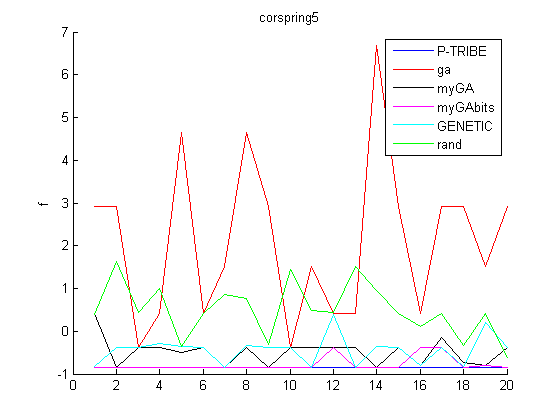Now the upper bound is raised to 10, so that the bounds are now -5 to 10, with the same solution of repeating 5's. Here you can see that with 3 variables MATLABs GA is considerably worse than all other methods, even including random guessing.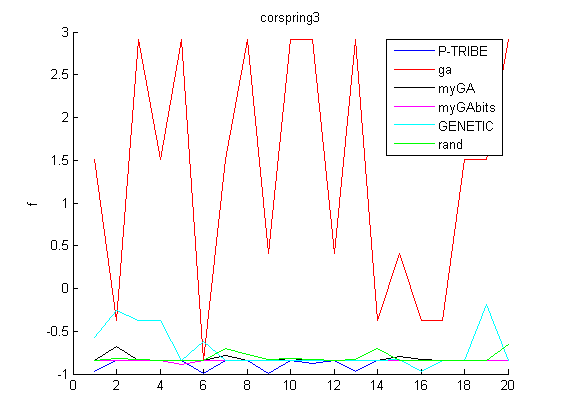Now the number of variables is changed to 10 with the same bounds and the same optimum solution. MATLABs GA has done better this time with my P-TRIBE and my GAbits also doing fairly well.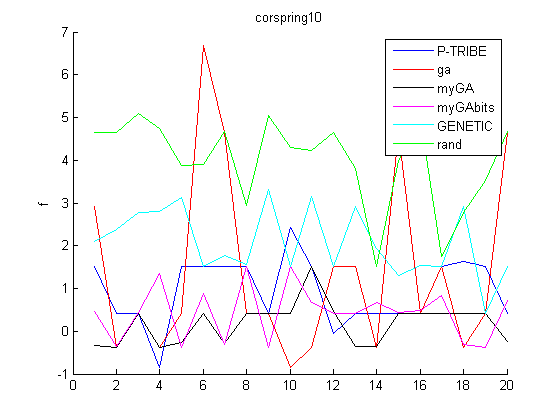Optimum Value vs. # of Variables

The previous plots all showed the f value (y-axis) over several different runs (x-axis). These plots take the average of all of these runs and plot the average f value (y-axis) vs. the # of variables (x-axis). In general MATLABs GA is certainly better than my methods, so I've got plenty of work to do if I want to try to beat their method. I think that the two biggest causes might be the following:

1) I set MATLABs GA to have the same number of function calls as my algorithms but it sometimes seems to disobey this limit that I set. Therefore with the higher numbers of variables MATLABs GA has an unfair advantage because it makes additional function calls.

2) My functions do repeat calls on the same point when the function value is already known so this inflates the number of function calls in my methods.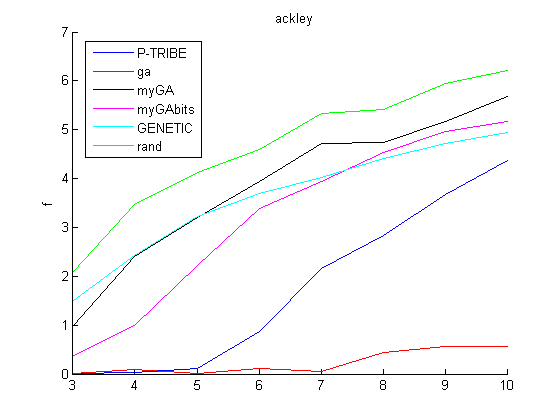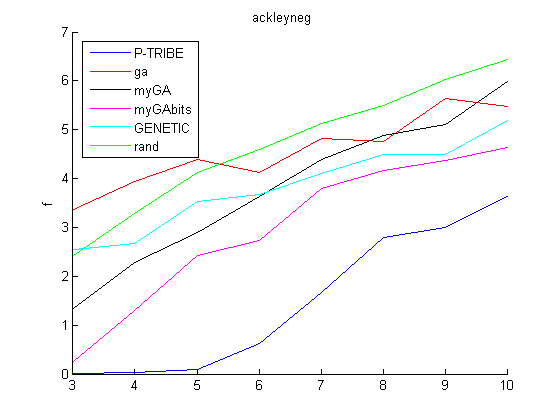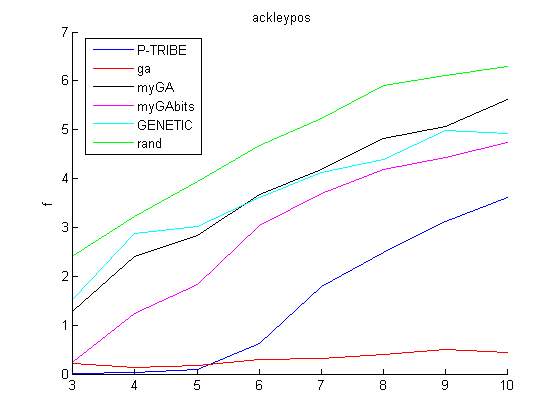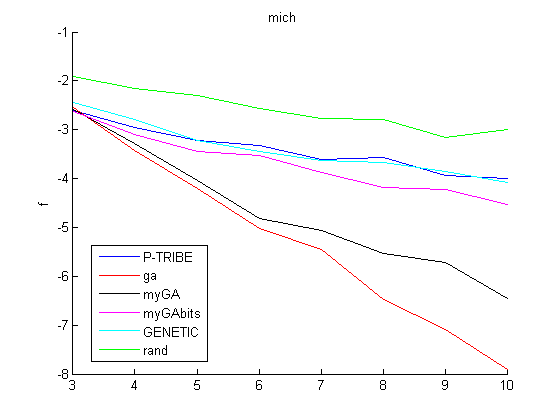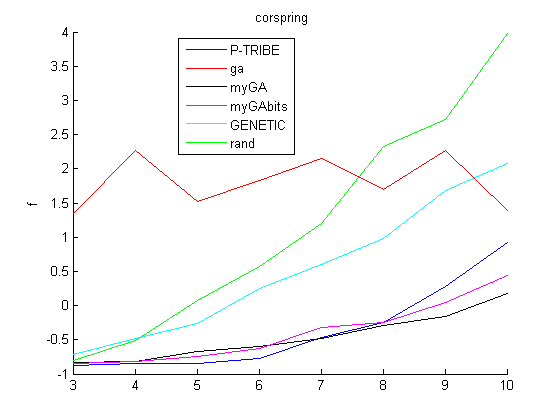Distribution of Solution Points

Here you can see a distribution of the solution points in one of the planes in the 3 variable space with the Corrugated Spring Function. Each dot in this plot represents the final solution point found by the optimization method. It is pretty clear that MATLABs GA never really leaves the center 1/3 of x values (x values of 0 to 5). All of the other methods have settled near the (5,5) area.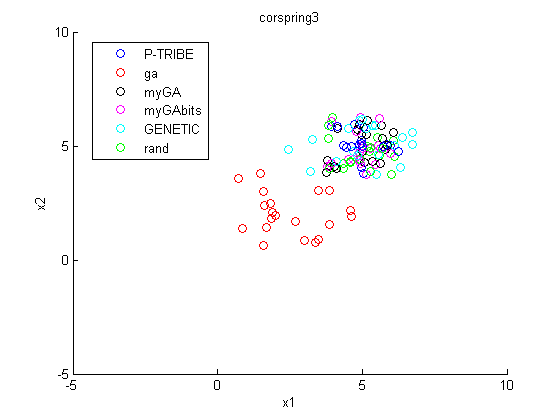zoomed in...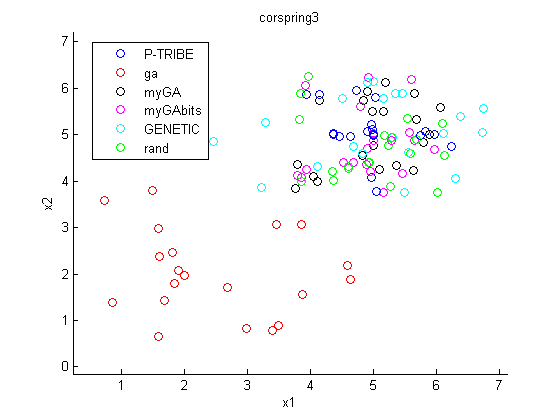Now back to the Ackley Function, you can see in these images of various zoom levels that the different optimization methods vary significantly in how often they ended up at the same point. The bounds were -5 to 5 and this first image is zoomed in to show only about -1 to 1. This zoom level caught nearly 100% of all of the points.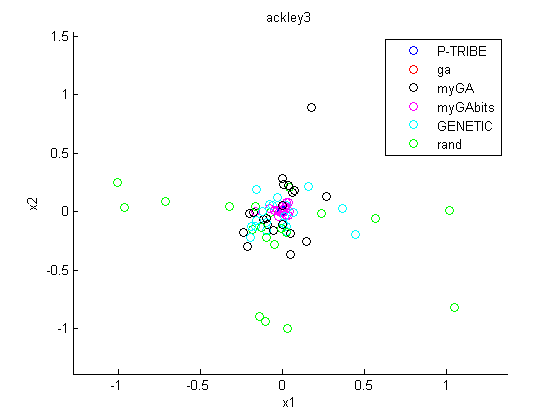After zooming in considerably you can now see that my P-TRIBEs and MATLABs GA always finish very close to zero, whereas the other methods are much more spread out.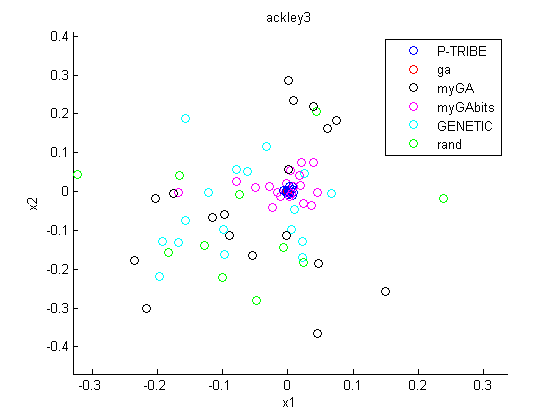At this zoom level it is clear that MATLABs GA does a very good job at getting as close to the optimum solution as possible. This is something that I need to work on to improve my methods.